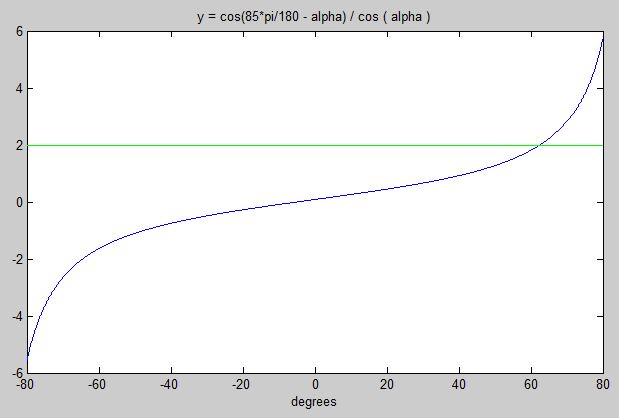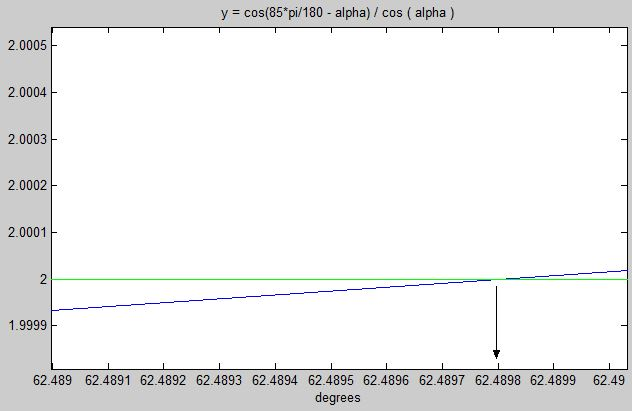Solve the following simultaneous equationsxenium used Ask the Experts™
on
hi, I'm breaking my head, i think the following is possible, 4 unknowns, 4 equations, can anyone help?

1) alpha + beta = 85 degrees
2) q * cos ( beta) = 6
3) cos(alpha) =3/q
4) b^2 = q^2 -9

Or perhaps one of the equations is derived from the others...

This is for some cardboard box design.

Thanks!
Comment
Watch Question

Do more withEXPERT OFFICE® is a registered trademark of EXPERTS EXCHANGE®

Commented:
change the degrees in 1 to radians

Commented:
I'm looking for an algebraic solution. You can work it in either degrees or radians that shoudl not make any difference.

Commented:
A first step could be use (1) to eliminate beta from (2)

q * cos (85 - alpha) = 6

...

Commented:
85 degrees = 1.48 radians

solve 4 for q
solve 1 for alpha

put results in 2 and 3
you now have two equations in two unknowns ( still messy but at least some progress)

Commented:
First 3 equations have only 3 unknowns, so don't consider equation 4 at first.
I would start by putting (3) in the same form as (2); i.e., multiply both sides by q.
You can then divide (2) by (3) to eliminate the q --> equation (5).

(1) and (5) are 2 equations in 2 unknowns (alpha, beta).
beta = 85 degrees - alpha
Plug beta into (5) and you have one equation in one unknown, i.e., alpha.

Commented:
>> You can work it in either degrees or radians that shoudl not make any difference.
You are right. It doesn't make a difference:
http://www.wolframalpha.com/input/?i=cos+(+85+degrees+-+alpha)%2F+cos+(+alpha+)+%3D+2
http://www.wolframalpha.com/input/?i=cos+(+85+*+pi%2F180+-+alpha)%2F+cos+(+alpha+)+%3D+2

Commented:
I can get it down to one equation in one unknown without much trouble, but then I'm stuck on solving it because of the trigonometry...

Commented:
Once you solve for alpha, then (1) gives you beta easily.
Either (2) or (3) gives you q.
Once you solve for q, then (4) gives you b easily.

Commented:
...looks like wolfram may help...

Commented:
>> I can get it down to one equation in one unknown without much trouble, but then I'm stuck on solving it because of the trigonometry...

Then why didn't you just ask that question, "one equation in one unknown" instead of giving us 4 equations?

Commented:
It doesn't matter what i start solving for.. i get stuck

Commented:
Don't you just need an answer? Why do you need a step-by-step solution (which wolfram says they can provide by going "Pro")?

Once getting one equation in one unknown, you can use plot utilities (or even by hand depending upon the number of precision required) to determine intersections of graphs.

Commented:
well the one equation looks very ugly. but some maybe less ugly than others depending how you proceed. 4 equations looks neat at least.

Commented:
I'm assuming the equations are solvable algebraically.. but i could be wrong. Some info here would be useful, my maths is rusty..

Commented:
example one equation, find b:

sqrt ( b^2 + 9 ) * cos(85 - acos(3 / sqrt ( b^2 + 9 ) )) = 6

Commented:
Does anyone have wolfram pro to check this? I'm getting "Standard computation time exceeded"

Commented:
It cannot be done. You are one equation short.

divide 2 by 3. That eliminates q.
b and q now appear only in 4.  (1 , 2, and 3 will allow you to find alpha and beta,leaving only 4 for both b and q.)

Commented:
aburr, if it can't be done then my one equation in one would be wrong.. but apart from being messy it seemed like a straightforward sequence of substitution...

...unless solving my one equation just gives b=b

hmmm

Commented:
1, 2, and 3 are completely independent of b and q. As far as they are concerned, b and q can be anything. They tell you nothing about b and q, so you have one equation in two unknowns.

Commented:
2) q * cos ( beta  ) = 6
3) q * cos ( alpha ) = 3

if you solved for alpha, then from (3) you know q.
But, if you solved for alpha, then you now know beta, and then you can solve for q using (2):

<<EDIT>>
We solved for this equation using Wolfram:
cos ( beta  ) / cos ( alpha ) = 2
alpha1 = 1.09065
alpha2 = -2.05094
which gives us beta:
beta1 =  0.3929
beta2 =  3.5345

Commented:
Thanks for your inputs. I think I need to sleep on this one...

Commented:
(Note: I edited my previous post since I had to also sleep on it in a power nap.)
We have:
alpha1 = 1.09065
alpha2 = -2.05094
which gives us beta:
beta1 =  0.3929
beta2 =  3.5345

We can now compute q for these four values of alpha and beta:
Equation (2):
>> q11 = 6/cos ( beta1 )
q11 =
6.4948
>> q12 = 6/cos ( beta2 )
q12 =
-6.4948

Equation (3):
>> q21 = 3/cos(alpha1)
q21 =
6.4948
>> q22 = 3/cos(alpha2)
q22 =
-6.4948

Commented:
equation (4)
>> b = sqrt(q11^2 - 9)
b =
5.7605

(Another solution is: b = -5.7605)

Commented:
As for solving this equation for alpha:
cos ( 85 * pi/180 - alpha)/ cos ( alpha ) = 2
I am tempted to avoid initially trigonometry manipulations, and instead would start with Euler's formula and see where that leads.
https://en.wikipedia.org/wiki/Euler%27s_formula#Relationship_to_trigonometry

BTW - as you noticed, the Wolfram solution indicates that there are an infinite number of solutions when solving for alpha. Depending upon what the four equations are modeling, you have to decide which solution is pertinent for your needs.
Mechanical Engineer
Most Valuable Expert 2013
Top Expert 2013
Commented:
I set the problem up in Excel, then used the Solver add-in to vary the values of alpha, beta, b & q until it found a solution. In setting the problem up, I rewrote the four equations so the right side equaled 0. Solver was then asked to minimize the sum of the squares of the right sides of each equation.

Constraints:
alpha between 0 and 85 degrees
beta between 0 and 85 degrees

Results:
alpha      62.5167201
beta      22.48327471
b      5.757738712
q      6.492426432
4EquationsQ28929349.xlsx
Commented:
Since you were wondering how to get an analytic solution, I have asked the question on getting the steps in solving the following equation that I noted in http:#a41483890
cos ( 85 * pi/180 - alpha)/ cos ( alpha ) = 2
Here is the question:
http://www.experts-exchange.com/questions/28929373/How-to-solve-a-trigonometry-equation.html

In summary, here are the numerical results of your four equations:
alpha = +1.09065 or -2.05094
beta  = +0.3929  or +3.5345
q     = +6.4948  or -6.4948
b     = +5.7605  or -5.7605
Commented:
In http:#a41483830 I wrote that you can use plot utilities to determine intersections of graphs.

Here is what I had in mind (which is consistent with the Wolfram solution) showing the expression from -80 to +80 degrees.Zooming in to where y = 2, we see that alpha = 62.4898 degrees * pi/180 = 1.09065 radians which is what was shown above.Not sure why byundt's solution differs a little. My utilities were using 64-bit precision. Maybe byundt's solution used 32-bit, or possibly stopped short when a reasonable threshold was reached.

Commented:
That's excellent thank you both for your help.

Commented:
I'd give 5000 points but there's a limit. Thank you all for shedding light on this tricky problem.

byundt, you make the subscription fee worth it on your own.

I may post a follow-up as I will be looking for a generic solution on google sheets.

Thanks again
Mechanical Engineer
Most Valuable Expert 2013
Top Expert 2013

Commented:
phoffric,
Excel uses 64-bit floating point numbers to achieve 1 part in 10^15 precision. That's the same degree of precision and accuracy as you were using.

Solver does quit when the solution converges to a certain degree. And that convergence would be measured against the sum of the squares of the residuals for the four equations. The default precision constraint is 1 part in a million, but you can set higher precision in the Solver...Options dialog. I assume that this is why there is a small difference between your graphical solution and the Solver results.

I didn't think xenium needed more than the default Solver accuracy, because this problem was "for some cardboard box design."

Commented:
Finally, an analytic solution..
Recall the equation to solve for alpha
Let C = 85 degrees
cos ( C- a ) / cos ( a ) = 2, where a ~ alpha
{ cos(C)*cos(a) + sin(C)*sin(a) } / cos(a) = 2
cos(C) + sin(C)tan(a) = 2

Now it is easy to isolate tan(a).
sin(C)tan(a) = 2 - cos(C)
tan(a) = [ { 2 - cos(C) } / sin(C) ]

Here is value of alpha:
alpha = arctan[ { 2 - cos(85) } / sin(85) ] = arctan[ { 2 - 0.087155743 } / 0.996194698 ]
alpha = arctan( 1.920151011 ) = 62.489843518 degrees

>> breaking my head, i think the following is possible
Your intuition was right; it is possible.
More importantly, no more need to break your head. :)

Do more withSubmit tech questions to Ask the Experts™ at any time to receive solutions, advice, and new ideas from leading industry professionals.